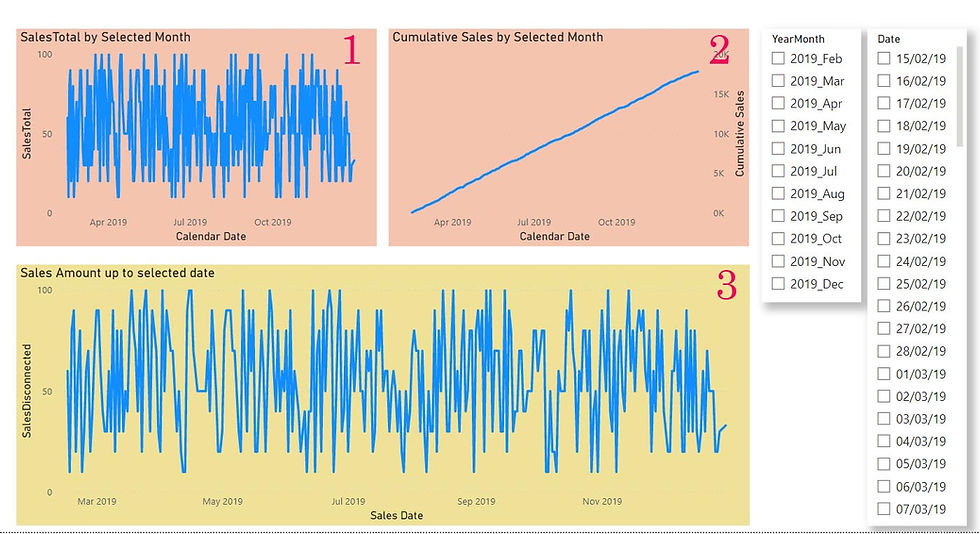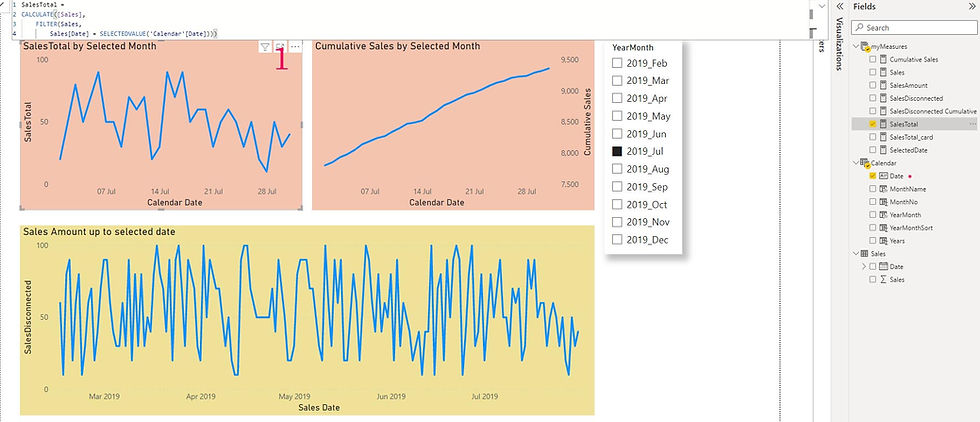top of page
Search
• Marek Vavrovic

# Disconnected Table / Dimension

Power BI Model:

I have two tables. Sales with transactions and dimension table for filtering.1. If no filter is applied.

Chart 1: shows whole period

Chart 2: shows whole cumulative period

Chart 3: shows whole period2. If Month is selected - filter is applied.

Chart 1: shows sales for selected period (July)

Chart 2: shows cumulative sales for selected period (July)

Chart 3: shows sales from beginning till selected period. (From February till July.)Measures:

Chart 1: Date attribute comes from the disconnected dimension.

1. calculate [Sales] measures.

Sales =

VAR SelectedFilter = SELECTEDVALUE('Calendar'[Date])

VAR Result =

IF (

OR (

ISFILTERED ( 'Calendar'[Date] ) = FALSE (),

MAX ( 'Sales'[DATE] ) > SelectedFilter

),

BLANK (),

CALCULATE (

[SalesAmount],

FILTER (

ALL ( 'Sales'[DATE] ),

'Sales'[DATE] <= SelectedFilter

&& YEAR ( 'Sales'[DATE] ) = YEAR ( SelectedFilter )

&& 'Sales'[DATE] <= MAX ( 'Sales'[DATE] ))))

RETURN

Result

2. calculate SalesTotal, a measure for the Chart2.

SalesTotal =

CALCULATE (

[Sales],

FILTER ( Sales, Sales[Date] = SELECTEDVALUE ( 'Calendar'[Date] ) )

)Chart 2: Date attribute comes from the disconnected dimension.

SalesAmount = SUM(Sales[Sales])

2.

Cumulative Sales =

CALCULATE (

[SalesAmount],

FILTER ( Sales, Sales[Date] <= MAX ( 'Calendar'[Date] ) )

)

You can replace MAX ( 'Calendar'[Date] ) ) with SELECTEDVALUE ( 'Calendar'[Date] ) )Chart 3: Date attribute comes from the Sales table.

SalesDisconnected =

VAR MaxFilteredYear =

YEAR ( MAX ( 'Calendar'[Date] ) )

VAR MaxFilteredDate =

MAX ( 'Calendar'[Date] )

VAR Result =

CALCULATE (

SUM ( Sales[Sales] ),

FILTER (

Sales,

MAX ( Sales[Date] ) <= MaxFilteredDate

&& YEAR ( Sales[Date] ) = MaxFilteredYear

)

)

RETURN

Result

if you click on this image, you can open the dashboard